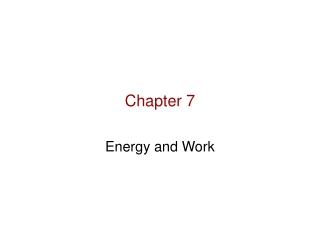Download PresentationChapter 7# Chapter 7 - PowerPoint PPT Presentation

Download Presentation##### Chapter 7

Download Policy: Content on the Website is provided to you AS IS for your information and personal use and may not be sold / licensed / shared on other websites without getting consent from its author. While downloading, if for some reason you are not able to download a presentation, the publisher may have deleted the file from their server.

- - - - - - - - - - - - - - - - - - - - - - - - - - - E N D - - - - - - - - - - - - - - - - - - - - - - - - - - -
##### Presentation Transcript

1. Chapter 7 Energy and Work

2. Goals for Chapter 7 • Overview energy. • Study work as defined in physics. • Relate work to kinetic energy. • Consider work done by a variable force. • Study potential energy. • Understand energy conservation. • Include time and the relationship of work to power.

3. Energy • Energy is conserved. • Kinetic Energy describes motion and relates to the mass of the object and it’s velocity squared. • Energy on earth originates from the sun. • Energy on earth is stored thermally and chemically. • Chemical energy is released by metabolism. • Energy is stored as potential energy in object height and mass and also through elastic deformation. • Energy can be dissipated as heat and noise.

4. Energy Transfer and Transformation Energy is transformed from one form to another, but it is not created or destroyed.

5. Mechanical energy—the energy associated with motion, position, and deformation of objects. Kinetic Energy. Elastic Potential Energy. Gravitational Potential Energy.

6. Energy can be dissipated by heat (motion transferred at the molecular level. This is referred to as dissipation.

7. WORK

8. What is “work” as defined in Physics? • Formally, work is the product of a constant force F through a parallel displacement s. W is N.m W = F.s 1 Joule (J) = (1N.m)

9. Forces applied at angles must be resolved into components. Parallel component = along the displacement

10. W > 0 W < 0 W = 0

11. Sliding on a ramp

12. Work done be several forces

13. Work done be several forces

14. Work Done by a Varying Force

15. Work Done by a Varying Force

16. Energy may be stored in compressed springs.

17. Work and Kinetic Energywork-energy theorem W = Kf - Ki

18. Work done be several forces Vi = 2.0 m/s

19. Gravitational Potential Energy • U = mgh • Independently of the path

20. Sliding on a ramp Vf ? M= 100kg, angle = 30 degrees, h =3m

21. Uel = (1/2) kx2

22. Work-Energy Theorem Wtotal = Kf – Ki • Conservatives force Kf + Uf = Ki + Ui • Non-conservative forces Kf + Uf = Ki + Ui + Wother • Power Pav = ΔW/Δt The unit is Watt (J/s)

23. A 1.50 kg book is sliding along a rough horizontal surface. At point A it is moving at 3.21m/s  , and at point B it has slowed to 1.25 m/s . How much work was done on the book between A and B ? If -0.750J of work is done on the book from B to C, how fast is it moving at point C? How fast would it be moving at C if +0.750 of work were done on it from B to C?

24. For its size, the common flea is one of the most accomplished jumpers in the animal world. A 2.0 mm  long, 0.50 mg  critter can reach a height of 20.0 cm  in a single leap. Neglecting air drag, what is the takeoff speed of such a flea? Calculate the kinetic energy of this flea at takeoff and its kinetic energy per kilogram of mass. If a 65.0 Kg , 2.00 m -tall human could jump to the same height compared with his length as the flea jumps compared with its length, how high could he jump, and what takeoff speed would he need?

25. A 1.50  kg box moves back and forth on a horizontal frictionless surface between two different springs, as shown in the accompanying figure. The box is initially pressed against the stronger spring, compressing it 4.00  cm, and then is released from rest. a) By how much will the box compress the weaker spring? b) What is the maximum speed the box will reach?

26. Problems with non-conservative forces

27. You slam on the brakes of your car in a panic and skid a distance d on a straight and level road. If you had been traveling twice as fast, what distance would the car have skidded under the same conditions? Use work energy theorem D) (1/2) d A) 4d B)2d C) d

28. Consider two frictionless inclined planes with the same vertical height. Plane 1 makes an angle of 25.0o with the horizontal, and plane 2 makes an angle of 55.0o with the horizontal. Mass m1 is placed at the top of plane 1, and mass m2 is placed at the top of plane 2. Both masses are released at the same time. At the bottom, which mass is going faster? A) m1 B) m2 C) neither (both same speed)

29. The stone in the accompanying figure can be carried from the bottom to the top of a cliff by various paths.   Which path requires more work? A) AC B) ABC C) same work for both paths